Processing ......FreeComputerBooks.com Links to Free Computer, Mathematics, Technical Books all over the World

Tea Time Numerical Analysis: Experiences in Mathematics
🌠 Top Free Unix/Linux Books - 100% Free or Open Source!
• Title: Tea Time Numerical Analysis: Experiences in Mathematics
• Author(s) Leon Q. Brin
• Publisher: CreateSpace (2014); eBook (3rd Edition, Southern Connecticut State University, 2021)
• Paperback: 276 pages
• eBook: PDF (375 pages)
• Language: English
• ISBN-10: 1502365820
• ISBN-13: 978-1502365828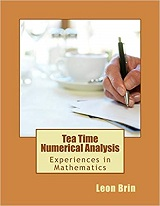Book Description

This textbook was born of a desire to contribute a viable, free, introductory Numerical Analysis textbook for instructors and students of mathematics. The ultimate goal of this book is to be a complete, one-semester, single-pdf, downloadable textbook designed for mathematics classes.

The topical coverage in Tea Time Numerical Analysis is fairly typical. The book starts with an introductory chapter, followed by root finding methods, interpolation (part 1), numerical calculus, interpolation (part 2), and the second edition introduces a chapter on differential equations.

In keeping with the desire to produce a completely free learning experience, GNU Octave was chosen as the programming language for this book.

• N/A
Reviews, Ratings, and Recommendations: Related Book Categories: Read and Download Links:Similar Books:
•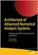Architecture of Advanced Numerical Analysis Systems

This unique open access book applies the functional OCaml programming language to numerical or computational weighted data science, engineering, and scientific applications. It's based on Owl, an OCaml-based numerical computing library.

•Numerical Algorithms: Computer Vision, Machine Learning, etc.

This book presents a new approach to numerical analysis for modern computer scientists, covers a wide range of topics - from numerical linear algebra to optimization and differential equations - focusing on real-world motivation and unifying themes.

•Numerical Methods and MATLAB Programming for Engineers

It covers the fundamentals while emphasizing the most essential numerical methods. Readers will enhance their programming skills using MATLAB to implement algorithms, discover how to use MATLAB to solve problems in science and engineering.

•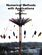Numerical Methods with Applications, 2nd Edition (Autar K Kaw)

This book entitled Numerical Methods with Applications is written primarily for engineering undergraduates taking a course in Numerical Methods. It offers a unique treatise to numerical methods which is based on a holistic approach and short chapters.

•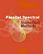Parallel Spectral Numerical Methods (Gong Chen, et al)

This book teaches the principals of Fourier spectral methods, their utility in solving partial differential equation and how to implement them in code. The programs will use Matlab and Fortran. A Python implementation of some of the Matlab programs is also provided.

•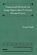Numerical Methods for Large Eigenvalue Problems (Yousef Saad)

This book is intended for researchers in applied mathematics and scientific computing as well as for practitioners interested in understanding the theory of numerical methods used for eigenvalue problems.

•First Semester in Numerical Analysis with Julia (Giray Okten)

This book presents the theory and methods, together with the implementation of the algorithms using the Julia programming language. The book covers computer arithmetic, root-finding, numerical quadrature and differentiation, and approximation theory.

•Numerical Analysis - Theory and Application (Jan Awrejcewicz)

This book is a book focused on introducing theoretical approaches of numerical analysis as well as applications of various numerical methods to either study or solving numerous theoretical and engineering problems.

•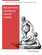High Performance Computing and Numerical Modelling

This textbook provides a step-by-step approach to numerical methods in engineering modelling. It provides a consistent treatment of the topic, from the ground up, to reinforce for students that numerical methods are a set of mathematical modelling tools.

•Numerical Computing with MATLAB (Cleve B. Moler)

This book is a lively textbook for an introductory course in numerical methods, MATLAB, and technical computing. It helps readers learn enough about the mathematical functions in MATLAB to use them correctly, and modify them appropriately, etc.

Book Categories
 :All CategoriesTop Free BooksRecent BooksMiscellaneous BooksComputer EngineeringComputer LanguagesComputer ScienceData Science/DatabasesJava and Java EE (J2EE)Linux and UnixMathematicsMicrosoft and .NETMobile ComputingNetworking and CommunicationsSoftware EngineeringSpecial TopicsWeb Programming
Other Categories